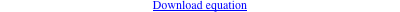too complicated to be written here. Click on the link to download a text file.X(2), X(3), X(3098), X(5092), X(7470), X(39162), X(39163), X(39164), X(39165), X(55177), X(55178) foci of the Steiner inellipse vertices of the Thomson triangle antipodes of points of K020 = pK(X6, X384) on (O) infinite points of pK(X6, P) with P = reflection of X(384) in X(376) vertices of the 1st and 2nd Neuberg triangles (centers of the Neuberg circles) Geometric properties :See Table 81 for general properties. K1334 is the cubic F(T) obtained when T = 4 / (3 - tan2ω) where ω is the Brocard angle. X(55177) and X(55178) are the Thomson isogonal conjugates of X(32) and X(39).